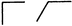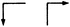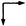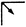International
Tables for
Crystallography
Volume A
Space-group symmetry
Edited by Th. Hahn

International Tables for Crystallography (2006). Vol. A. ch. 1.4, p. 7

## Section 1.4.2. Symmetry planes parallel to the plane of projection

Th. Hahna*

aInstitut für Kristallographie, Rheinisch-Westfälische Technische Hochschule, Aachen, Germany
Correspondence e-mail: hahn@xtal.rwth-aachen.de

### 1.4.2. Symmetry planes parallel to the plane of projection

| top | pdf |

Symmetry planeGraphical symbolGlide vector in units of lattice translation vectors parallel to the projection planePrinted symbol
Reflection plane, mirror planeNone m
Axial' glide planelattice vector in the direction of the arrow a, b or c
Double' glide plane (in centred cells only)e
Diagonal' glide planen
Diamond' glide plane§ (pair of planes; in centred cells only)in the direction of the arrow; the glide vector is always half of a centring vector, i.e. one quarter of a diagonal of the conventional face-centred cell d
The symbols are given at the upper left corner of the space-group diagrams. A fraction h attached to a symbol indicates two symmetry planes with heights' h andabove the plane of projection; e.g.stands forand. No fraction meansand(cf. Section 2.2.6).
For further explanations of the double' glide plane e see Note (iv)below and Note (x)in Section 1.3.2.
§Glide planes d occur only in orthorhombic F space groups, in tetragonal I space groups, and in cubic I and F space groups. They always occur in pairs with alternating glide vectors, for instanceand. The second power of a glide reflection d is a centring vector.